## 深度学习：循环神经网络原理

5804 字 · 207 阅读 · 2023 年 06 月 03 日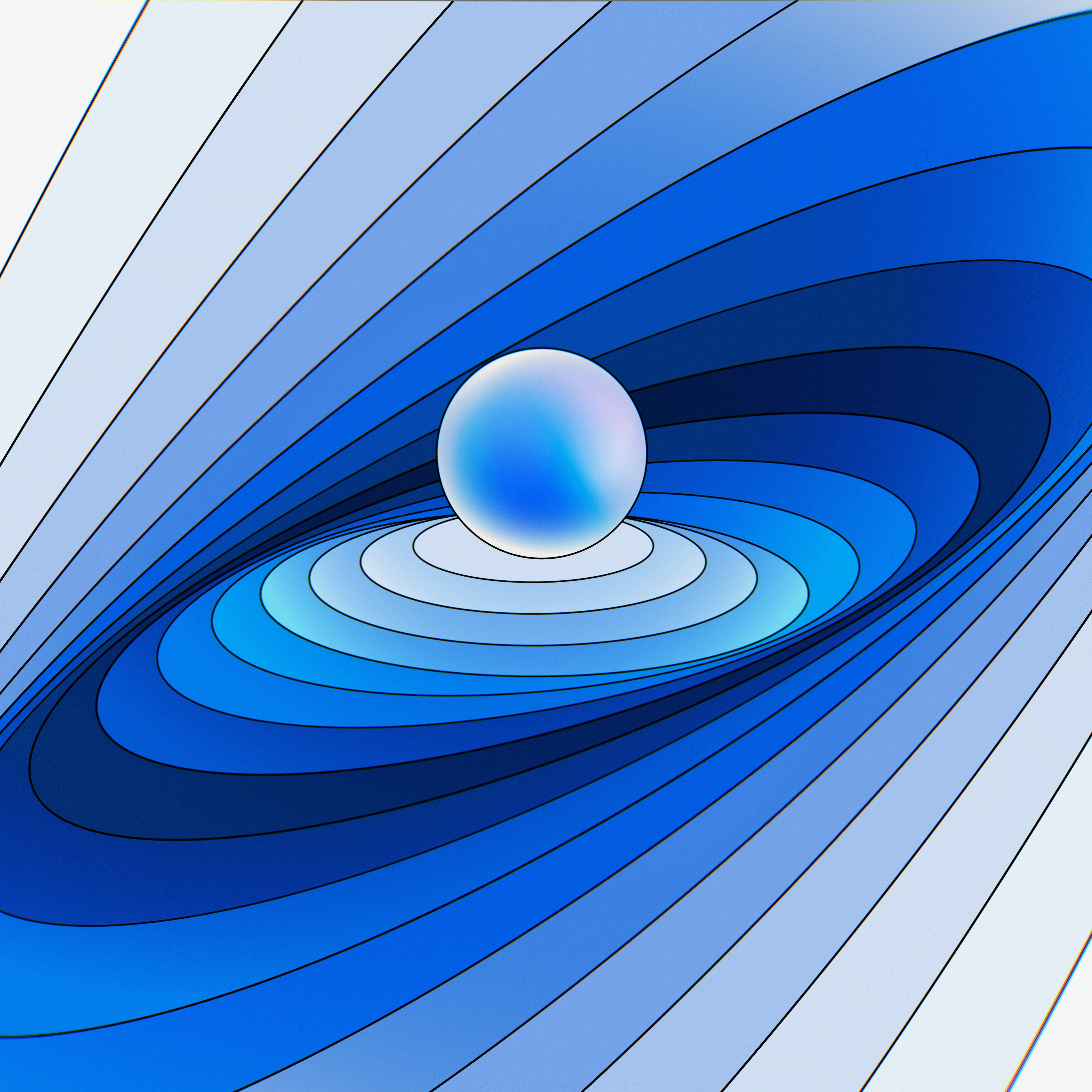• 序列模型介绍
• 简单循环神经网络
• LSTM 长短期记忆模型
• GRU 门控循环单元

### 自然语言处理介绍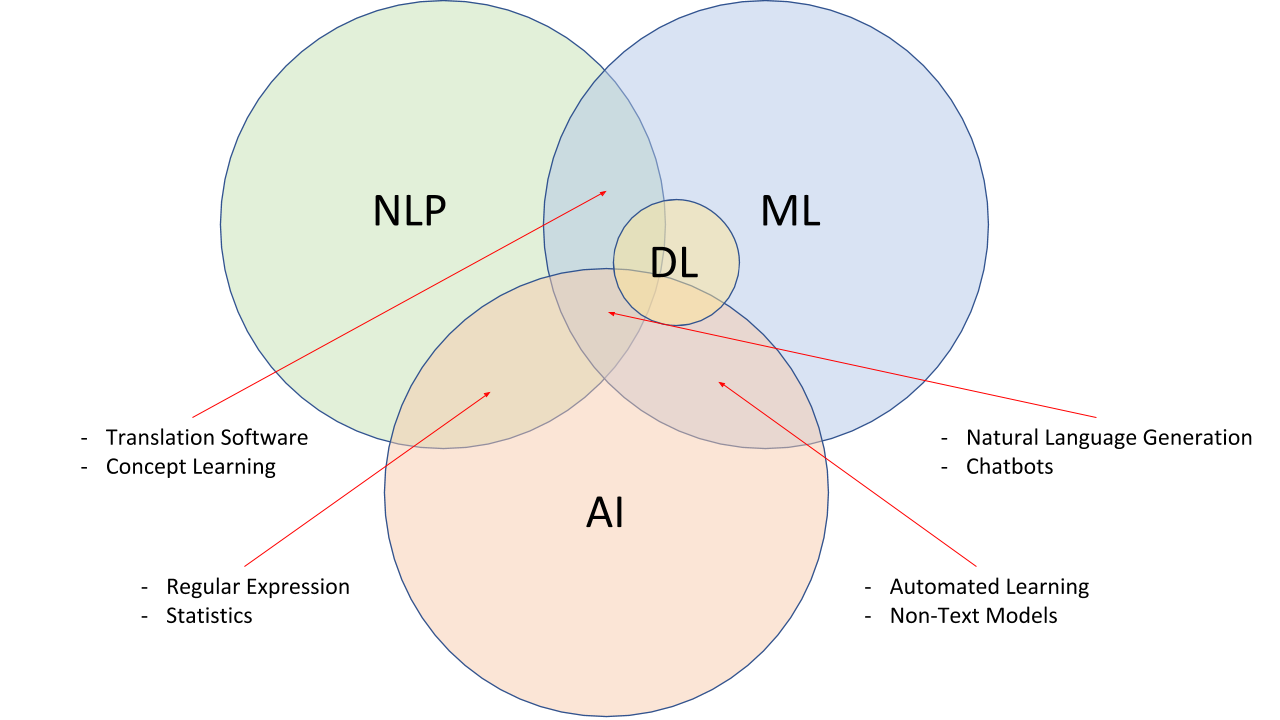• 文本规划：完成结构化数据中的基础内容规划。
• 语句规划：从结构化数据中组合语句来表达信息流。
• 实现：产生语法通顺的语句来表达文本。

• 信息检索：对大规模文档进行索引。
• 语音识别：识别包含口语在内的自然语言的声学信号转换成符合预期的信号。
• 机器翻译：将一种语言翻译成另外一种语言。
• 智能问答：自动回答问题。
• 对话系统：通过多回合对话，跟用户进行聊天、回答、完成某项任务。
• 文本分类：将文本自动归类。
• 情感分析：判断某段文本的情感倾向
• 文本生成：根据需求自动生成文本
• 自动文摘：归纳，总结文本的摘要。

### 序列模型介绍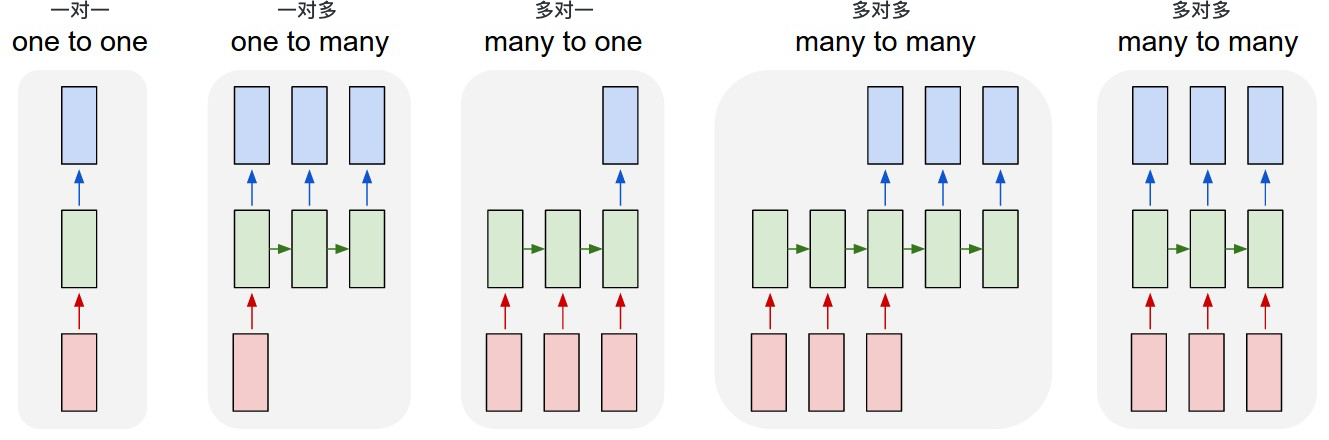• 一对一模型：我们之前的模型，比如全连接神经网络，就是一对一的结构。我们给它一个输入，它能得到一个输出，而且不同输入间被视作了独立的关系，分别进行学习或者识别等任务。而现在我们要关注的，是后四幅图，也就是时序的模型。
• 一对多模型：根据模型的一个输出，可以用来预测一个序列。比如对于一个图像，输出一串字幕来描述它。
• 多对一模型：根据一个序列输入，从而预测一个值。比如根据用户在一家饭店的留言，判断用户对这家饭店的评价。那么，输入是一段话是一个序列输入，输出是 0 至 5 之间的一个数作为打分。
• 多对多有延迟：根据模型的序列输入，我们根据已有的输入，有延迟地输出一个序列。常见的任务比如翻译的学习任务，英文翻译成中文。模型需要等英文输入到一定程度后，才能翻译成中文，这样输入输出都为一个序列。
• 多对多无延迟：根据模型的序列输入，根据输入同步输出一个序列。常见的比如做天气预报，需要实时根据测得的温度、湿度等做出下雨概率的预测。但是，每一次预测其实也要考虑之前的一些序列输入，而不仅由这一时刻的输入所决定。

### 简单循环神经网络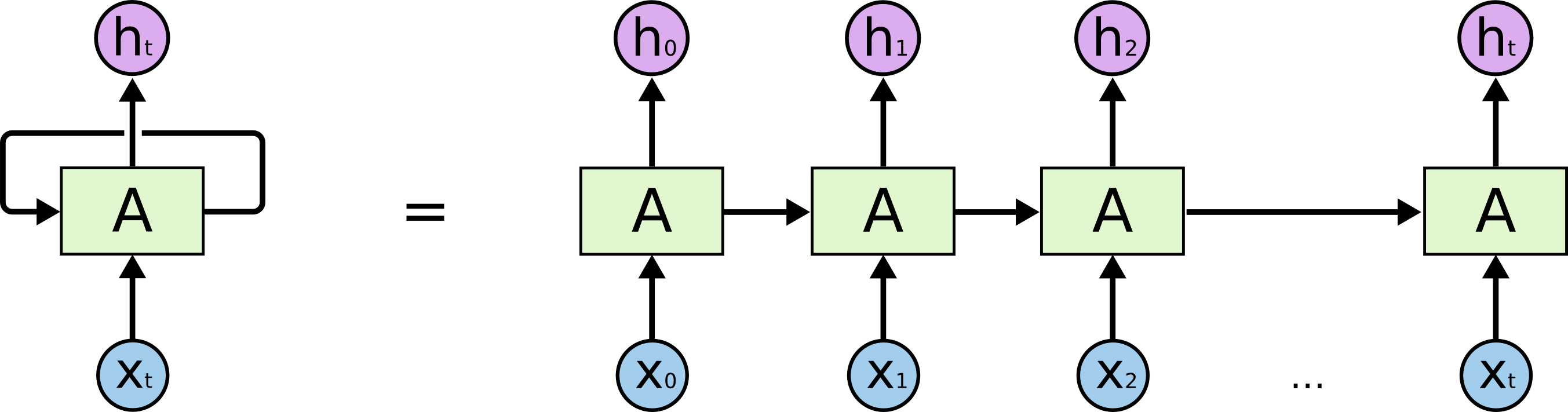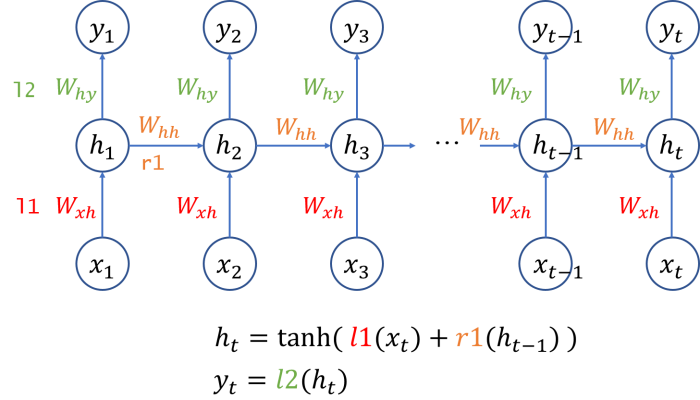$$h_2 = l_1(x_1) + r_1(h_1) \tag{1}$$

### LSTM 长短期记忆模型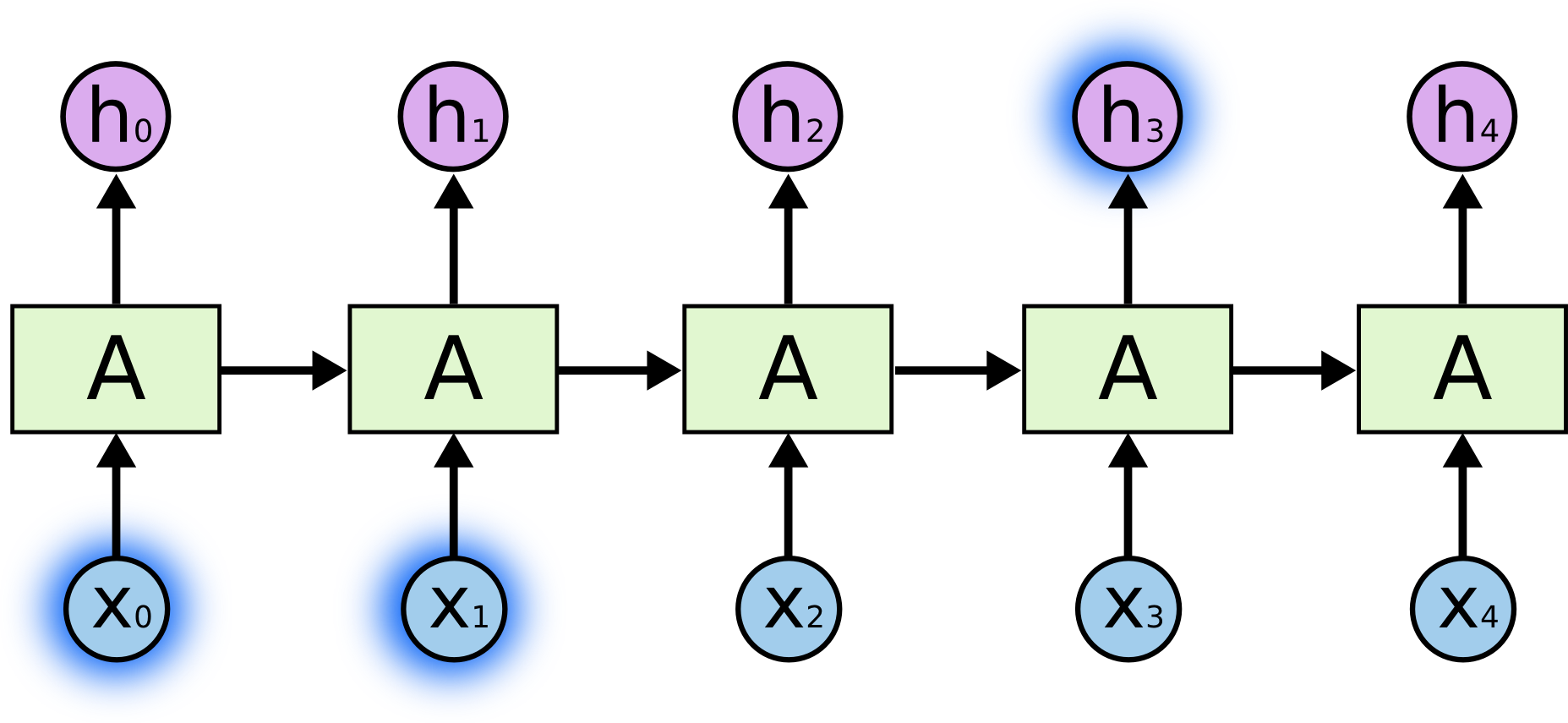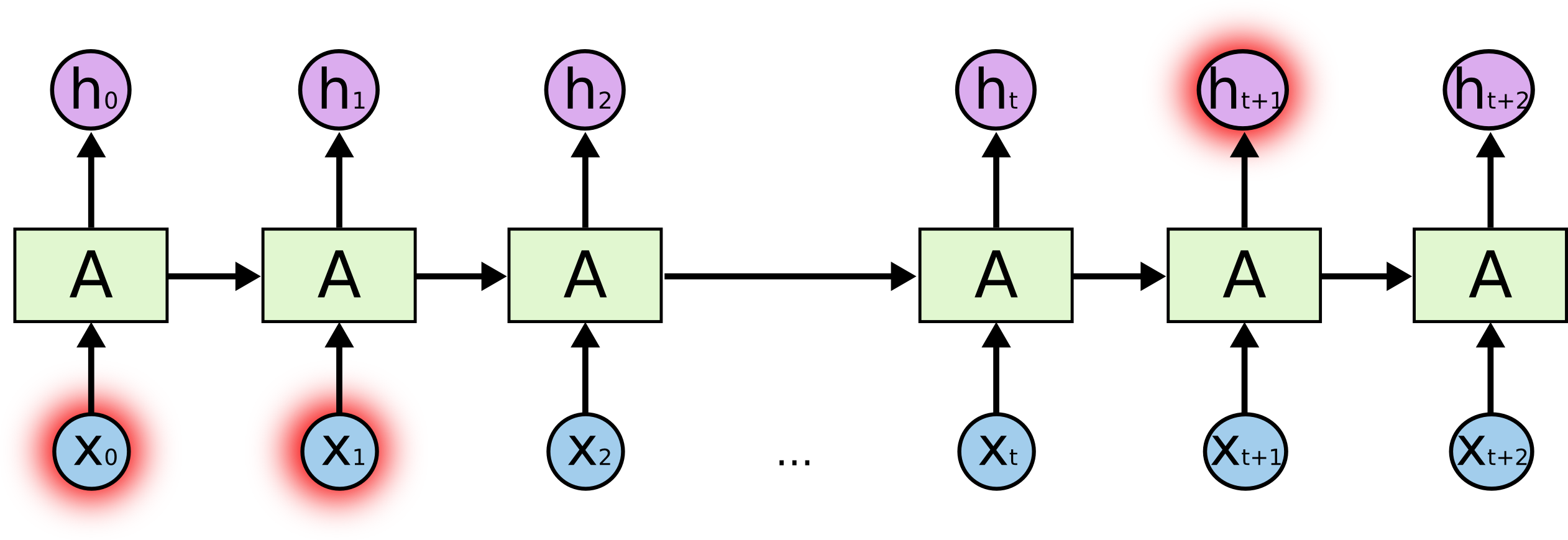LSTM 全称是 长短期记忆模型，是目前非常流行的循环神经网络元结构。它与一般的 RNN 结构本质上并没有什么不同，只是使用了不同的函数去计算隐藏层的状态。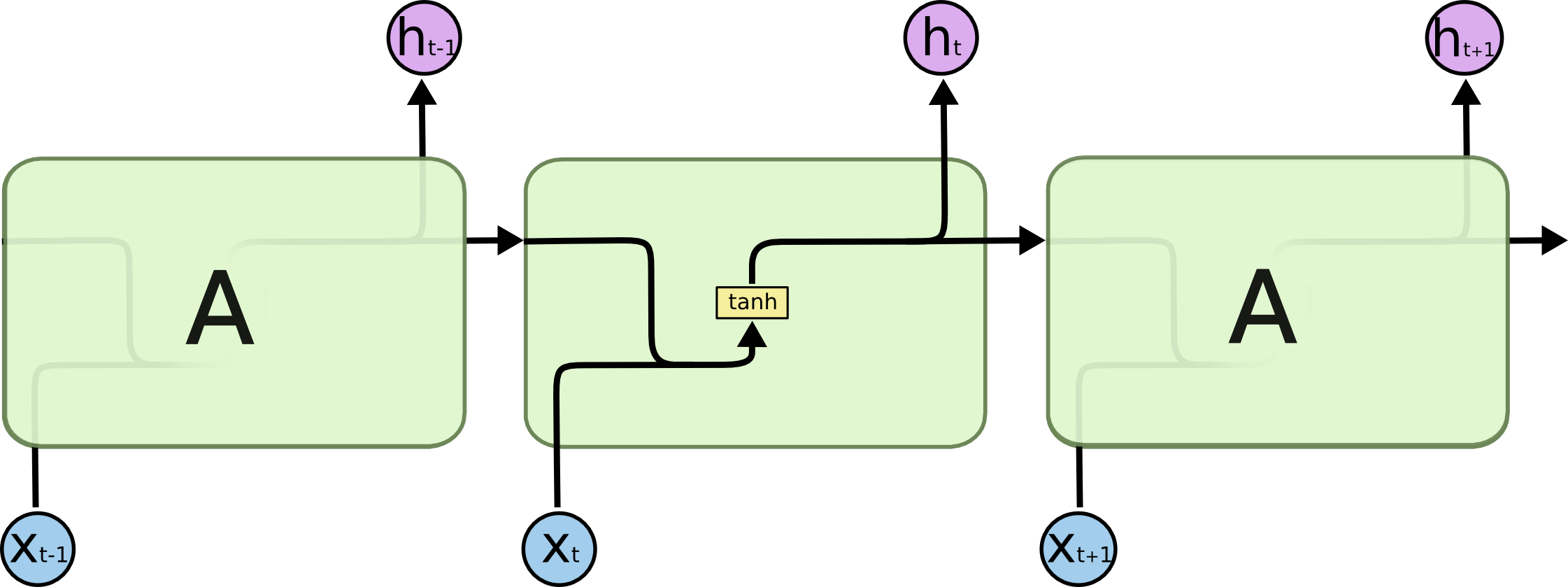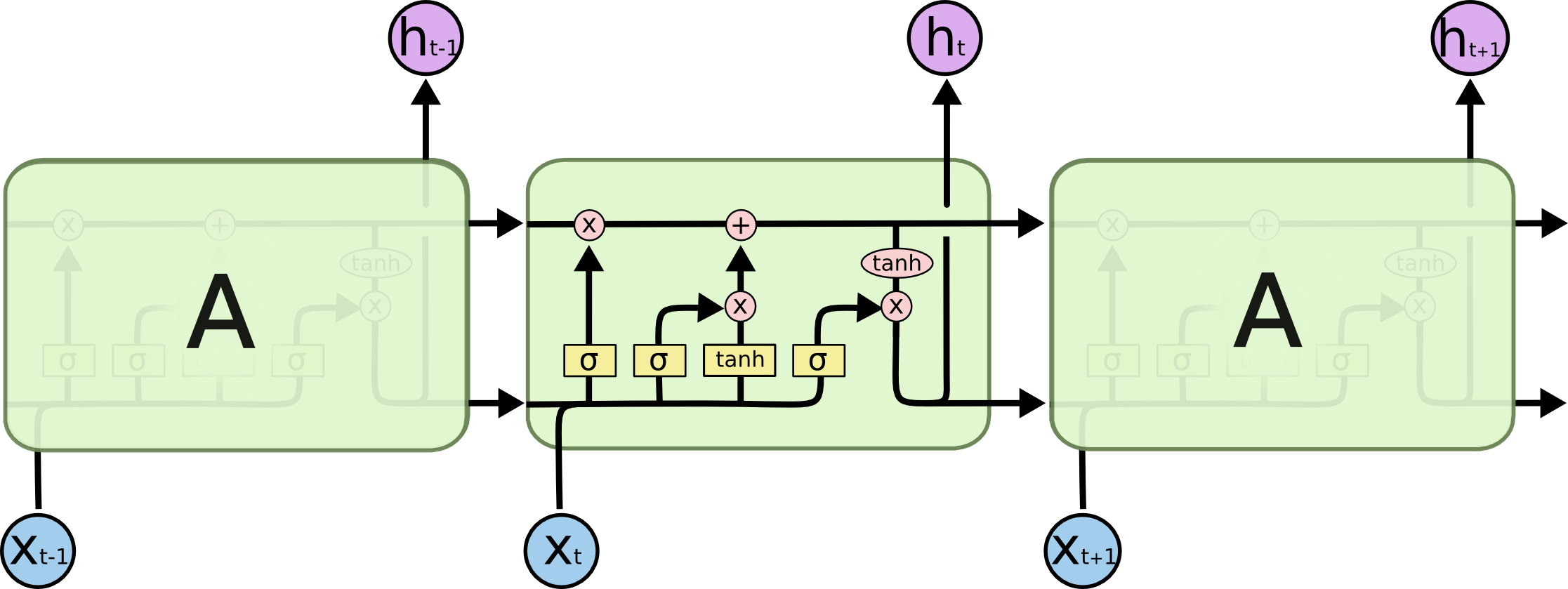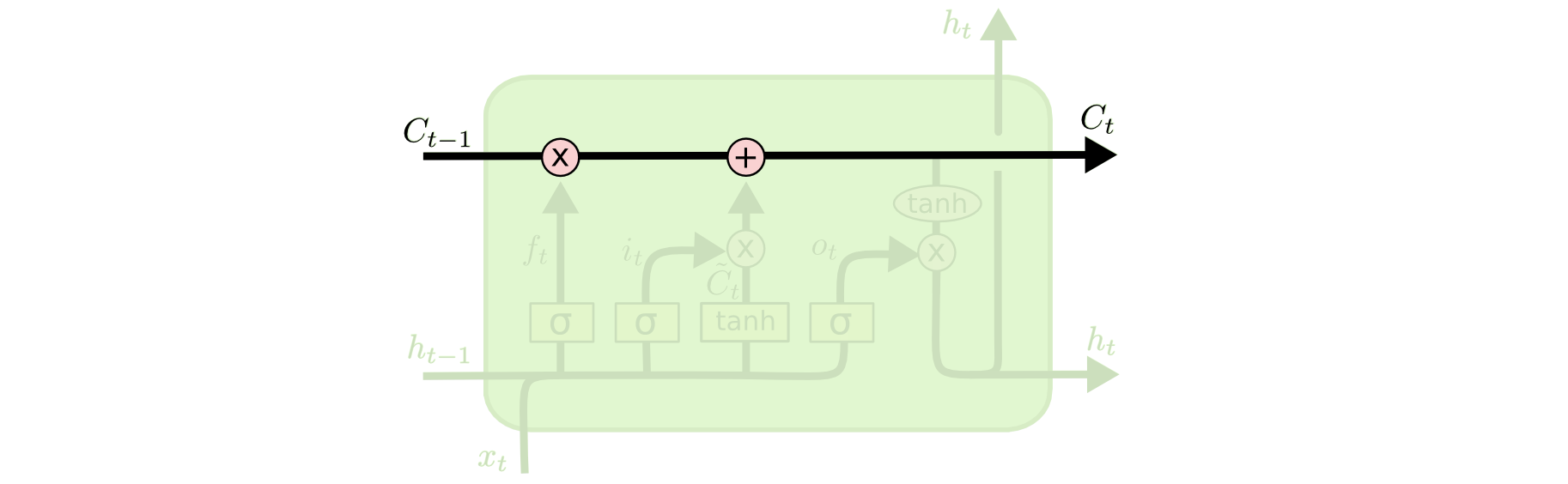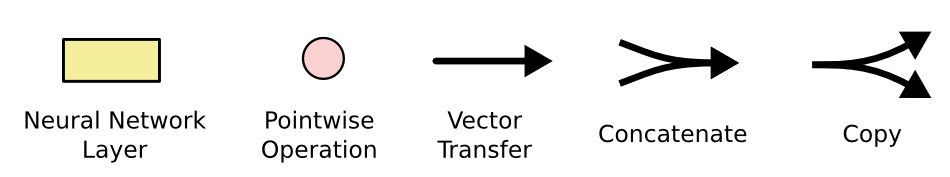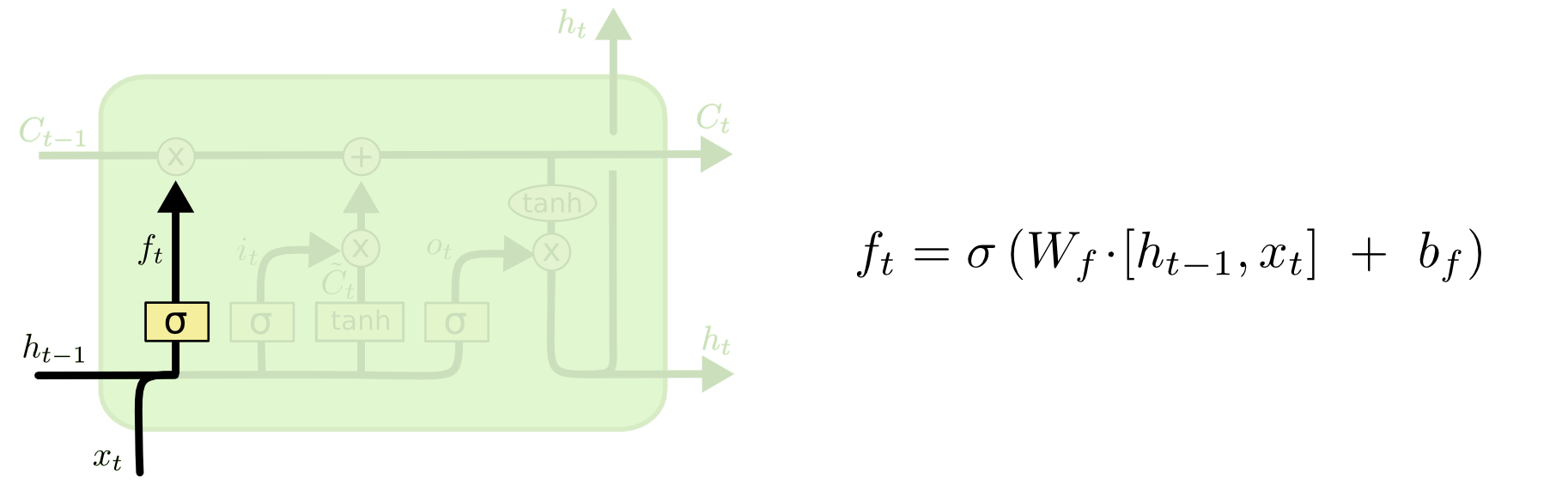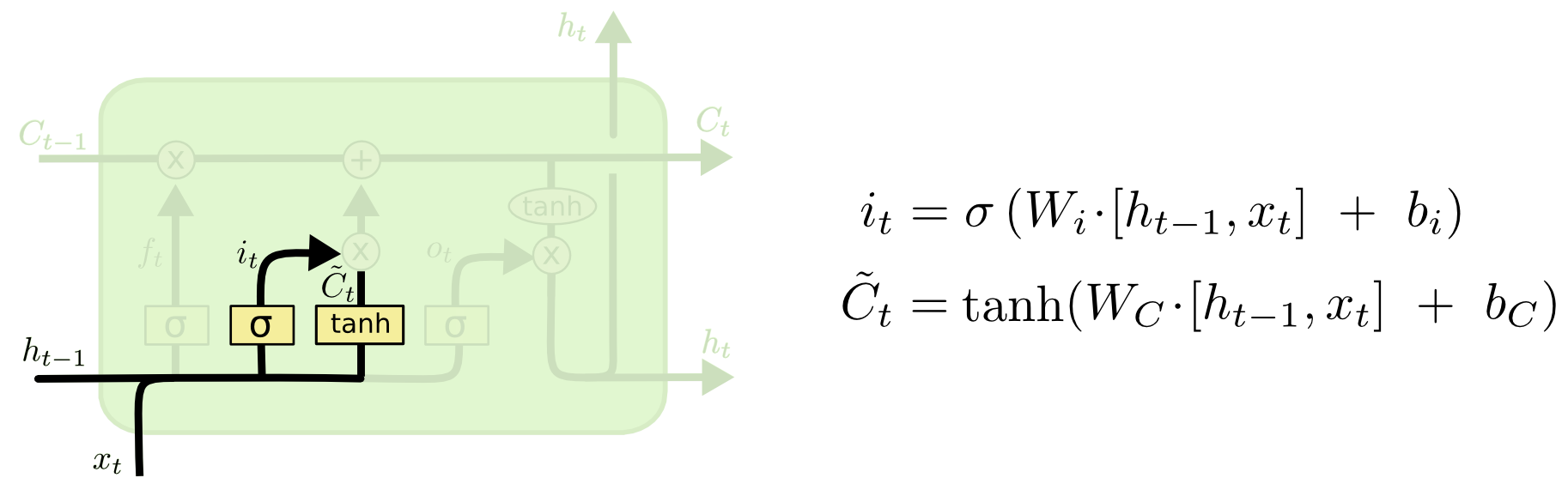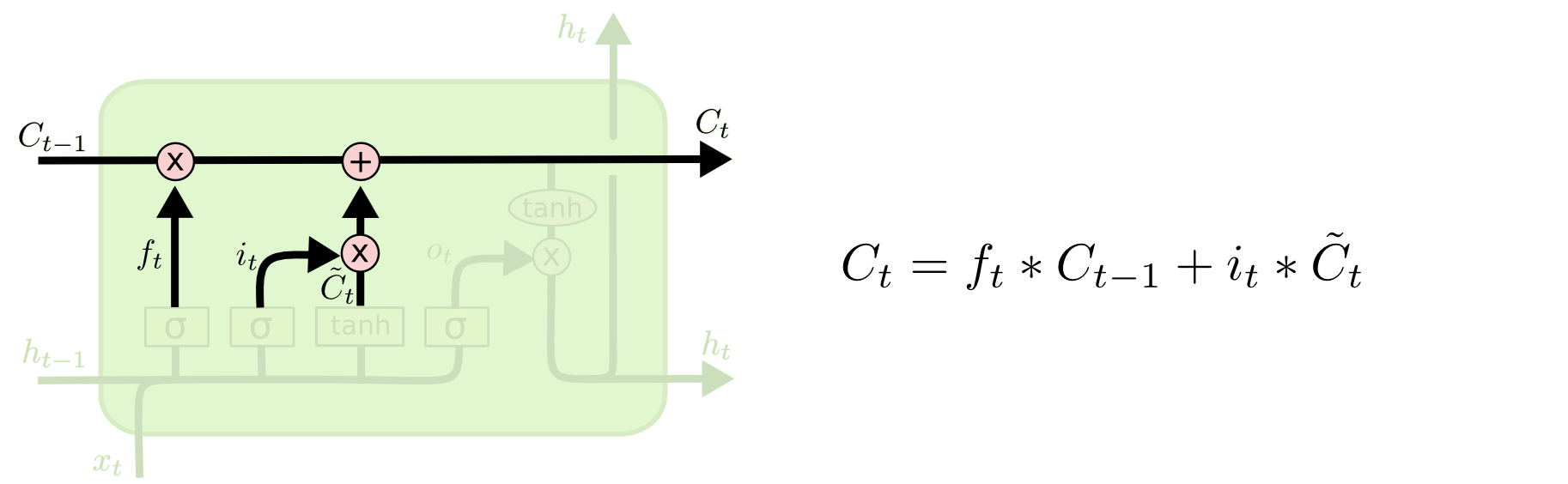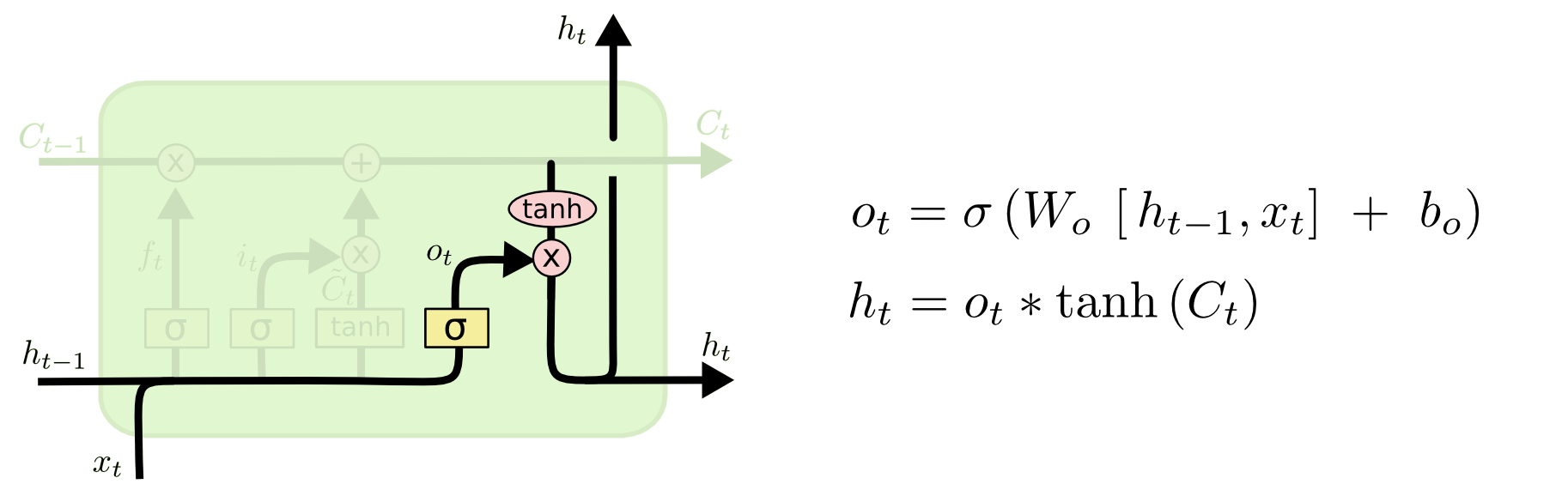$$f_{t}=\sigma(W_{f}\cdot x_{t}+U_{f}\cdot h_{t-1}+b_{f}) \tag{2}$$
$$i_{t}=\sigma(W_{i}\cdot x_{t}+U_{i}\cdot h_{t-1} +b_{i}) \tag{3}$$
$$\tilde{C}_{t}=\tanh(W_{c}\cdot x_{t}+U_{c}\cdot h_{t-1}+b_{c}) \tag{4}$$
$$o_{t}=\sigma(W_{o}\cdot x_{t}+U_{o}\cdot h_{t-1}+b_{o}) \tag{5}$$

$$C_{t}=f_{t}\cdot C_{t-1}+i_{t}\cdot \tilde{C}_{t} \tag{6}$$

$$h_{t} = o_{t}\cdot tanh({C}_{t}) \tag{7}$$

### GRU 门控循环单元

GRU 即 ↗ Gated Recurrent Unit。前面说到，为了克服 RNN 无法很好处理远距离依赖而提出了 LSTM，而 GRU 则是 LSTM 的一个变体。当然，LSTM 还有很多其它的变体。GRU 保持了 LSTM 的效果同时又使结构更加简单，所以它也非常流行。我们来看一下 GRU 的核心模块。$$r_{t}=\sigma(W_{r}\cdot x_{t}+U_{r}\cdot h_{t-1}+b_{r}) \tag{8}$$
$$z_{t}=\sigma(W_{z}\cdot x_{t}+U_{z}\cdot h_{t-1}+b_{z}) \tag{9}$$
$$\tilde{h_{t}}=tanh(W_{h}\cdot x_{t}+U_{h}\cdot h_{t-1}\cdot r_{t}+b_{h}) \tag{10}$$
$$h_{t}= z_{t}\cdot \tilde{h_{t}}+(1-z_{t})\cdot h_{t-1} \tag{11}$$

LSTM 还有很多的变种，比如 Gers, et al. (2000) 介绍的广受欢迎的 LSTM 变种添加了「门镜连接」。这意味着我们可以让门观察 Cell 状态。比如 Yao, et al. (2015) 提出的 Depth Gate RNN，又比如 Koutnik, et al. (2014) 提出的 Clockwork RNN 等等。就 GRU 和 LSTM 来说，GRU 的参数更少，因而训练稍快或需要更少的数据来泛化。另一方面，如果你有足够的数据，LSTM 的强大表达能力可能会产生更好的结果。

### 双向 RNN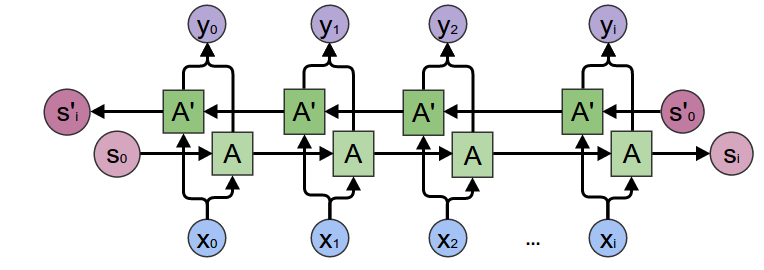### 深度 RNN### 系列文章Write a Comment
•• 写的真不错。请问transformer写了吗

• LSTM学习参数总结中的公式7格式错了

• 文末相关链接2「微软...」链接地址不对

• @Forrest Yang, 已经修正

• @huhuhang 公式(7)应为： $$h_{t}=o_{t} * \tanh \left(C_{t}\right)$$

• @Forrest Yang, 感谢，自动格式化工具把下划线识别成 md 标记处理成 * 了。😂